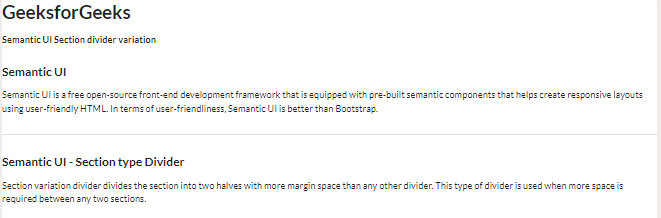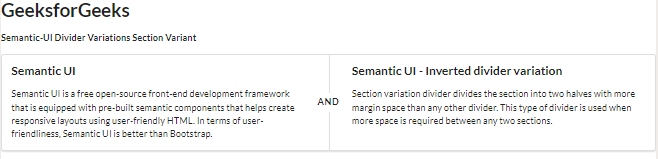Open in App
Not now

# Semantic-UI Divider Variations Section Variant

• Last Updated : 02 Feb, 2022

Semantic UI is a free open-source front-end development framework that is equipped with pre-built semantic components that helps create responsive layouts using user-friendly HTML.  It uses predefined CSS and jQuery to incorporate different frameworks.

In this article, let us discuss Section variation in the divider variations supported in Semantic UI. A divider is generally used to divide the content concisely into different sections.

The divider section variation divides the section into two halves with more margin space than any other divider. This type of divider is used when more space is required between any two sections. Section variation gives large margins to divide sections of web content.

Semantic-UI Divider Variations Section Variant Class:

• section: This class is used to divide the header from each other.

Syntax:

```<element class="ui header">....</element >
<element class="ui section divider">....</element>

Below examples illustrate the Semantic-UI Divider Variations Section Variant:

Example 1: In the below example, we can see a section divider variation using a horizontal divider, which divides two sections into sectioned vertical sections.

## HTML

 ` ` `<``html``> ` ` `  `<``head``> ` `    ``<``link` `href``= ` `"https://cdnjs.cloudflare.com/ajax/libs/semantic-ui/2.4.1/semantic.min.css"`  `          ``rel``=``"stylesheet"` `/>  ` ` ` ` `  `<``body``> ` `    ``<``h1``>GeeksforGeeks ` `    ``<``strong``>Semantic UI Section divider variation ` `    ``<``h3` `class``=``"ui header"``> Semantic UI ` `    ``<``p``> ` `        ``Semantic UI is a free open-source front-end ` `        ``development framework that is equipped with ` `        ``pre-built semantic components that helps create ` `        ``responsive layouts using user-friendly HTML. ` `        ``In terms of user-friendliness, Semantic UI is ` `        ``better than Bootstrap. ` `    `` ` ` `  `    ``<``div` `class``=``"ui section divider"``> ` `    ``<``h3` `class``=``"ui header"``>  ` `        ``Semantic UI - Section type Divider  ` `    `` ` `    ``<``p``> ` `        ``Section variation divider divides the section ` `        ``into two halves with more margin space than any ` `        ``other divider. This type of divider is used when ` `        ``more space is required between any two sections.  ` `    `` ` ` `  ` ` ` `  ``

Output:Semantic-UI Divider Variations Section Variant

Example 2: In the below example, we can see a section divider variation using a vertical divider, which divides two sections into sectioned vertical sections.

## HTML

 ` ` `<``html``> ` ` `  `<``head``> ` `    ``<``link` `href``= ` `"https://cdnjs.cloudflare.com/ajax/libs/semantic-ui/2.4.1/semantic.min.css"`  `          ``rel``=``"stylesheet"` `/>  ` ` ` `<``body``> ` `    ``<``h1``>GeeksforGeeks ` `    ``<``strong``>Semantic-UI Divider Variations Section Variant ` `    ``<``div` `class``=``"ui segment"``> ` `        ``<``div` `class``=``"ui two column very relaxed grid"``> ` `            ``<``div` `class``=``"column"``> ` `                ``<``h3` `class``=``"ui header"``> Semantic UI ` `                ``<``p``> ` `                    ``Semantic UI is a free open-source front-end  ` `                    ``development framework that is equipped with ` `                    ``pre-built semantic components that helps create ` `                    ``responsive layouts using user-friendly HTML.  ` `                    ``In terms of user-friendliness, Semantic UI is ` `                    ``better than Bootstrap. ` `                `` ` ` `  `            `` ` `            ``<``div` `class``=``"column"``> ` `                ``<``h3` `class``=``"ui header"``>  ` `                 ``Semantic UI - Inverted divider variation  ` `                `` ` `                ``<``p``> ` `                    ``Section variation divider divides the section  ` `                    ``into two halves with more margin space than any ` `                    ``other divider. This type of divider is used when ` `                    ``more space is required between any two sections. ` `                `` ` ` `  `            `` ` `        `` ` `        ``<``div` `class``=``"ui sectioned vertical divider"``>AND ` `    `` ` ` ` ` `  ``

Output:My Personal Notes arrow_drop_up
Related Articles